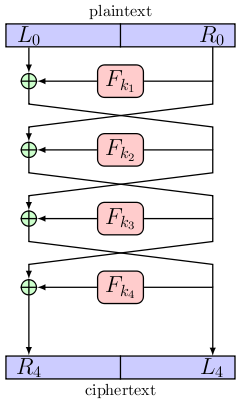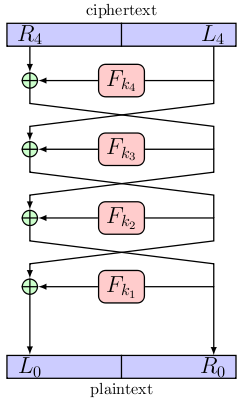# Feistel Cipher

A Feistel cipher/Feistel network is a class of block cipher which comprises several identical rounds, each round constructed from a function and a a swap.

The input block is split in the middle to give to equal sized sub-blocks. Let us call the initial left-hand side sub-block `L0`, and the initial right-hand side sub-block `R0`. Let the function `Fki` be the round function which uses the `i`th round key, `ki`. These round keys are generated by the key schedule which is unspecified. If we have `r` rounds, then for `1≤i<r`

```	```
Li = Ri-1
Ri = Li-1⊕Fki(Ri-1)
```
```

and for `i=r`

```	```
Lr = Rr-1⊕Fkr(Lr-1)
Rr = Lr-1
```
```

The figure below shows a Feistel network with four rounds being used to encrypt a plaintext message.The process of decryption is identical to the one for encryption as long as the round keys are used in reverse order. The figure below shows decryption.When compared to a substitution/permutation network we see that the Feistel network has an advantage in that it does not require that the round function have an inverse.

## References

L. R. Knudsen, M. J. B. Robshaw, The Block Cipher Companion. Springer, 2011.